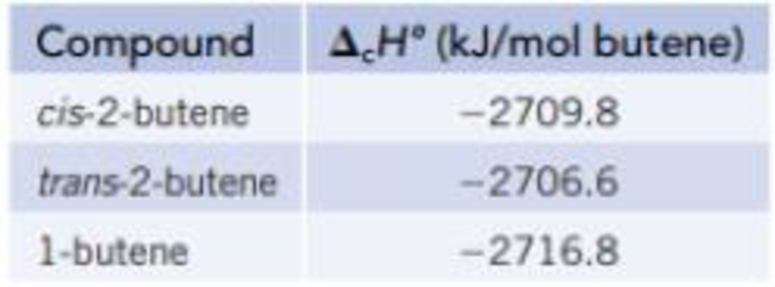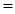# Isomers are molecules with the same elemental composition but a different atomic arrangement. Three isomers with the formula C 4 H 8 are shown in the models below. The enthalpy of combustion (Δ c H °) of each isomer, determined using a calorimeter, is as follows: (a) Draw an energy level diagram relating the energy content of the three isomers to the energy content of the combustion products, CO 2 ( g ) and H 2 O( ℓ ). (b) Use the Δ c H ° data in part (a), along with the enthalpies of formation of CO 2 ( g ) and H 2 O( ℓ ) from Appendix L, to calculate the enthalpy of formation for each of the isomers. (c) Draw an energy level diagram that relates the enthalpies of formation of the three isomers to the energy of the elements in their standard states. (d) What is the enthalpy change for the conversion of cis -2-butene to trans -2-butene?### Chemistry & Chemical Reactivity

9th Edition
John C. Kotz + 3 others
Publisher: Cengage Learning
ISBN: 9781133949640

#### Solutions

Chapter
Section### Chemistry & Chemical Reactivity

9th Edition
John C. Kotz + 3 others
Publisher: Cengage Learning
ISBN: 9781133949640
Chapter 5, Problem 111SCQ
Textbook Problem
12 views

## Isomers are molecules with the same elemental composition but a different atomic arrangement. Three isomers with the formula C4H8 are shown in the models below. The enthalpy of combustion (ΔcH°) of each isomer, determined using a calorimeter, is as follows:(a) Draw an energy level diagram relating the energy content of the three isomers to the energy content of the combustion products, CO2(g) and H2O(ℓ). (b) Use the ΔcH° data in part (a), along with the enthalpies of formation of CO2(g) and H2O(ℓ) from Appendix L, to calculate the enthalpy of formation for each of the isomers. (c) Draw an energy level diagram that relates the enthalpies of formation of the three isomers to the energy of the elements in their standard states. (d) What is the enthalpy change for the conversion of cis-2-butene to trans-2-butene?

(a)

Interpretation Introduction

Interpretation:

The energy level diagram relating the energy content of the three isomers has to be determined

Concept Introduction:

Heat energy required to raise the temperature of 1g of substance by 1K.Energy gained or lost can be calculated using the below equation.

q=C×m×ΔT

Where, q= energy gained or lost for a given mass of substance (m), C =specific heat capacity,ΔT= change in temperature.

The standard molar enthalpy of formation is the enthalpy change ΔfH0 is the enthalpy change for the formation of 1mol a compound directly from its component elements in their standard states. And is given by

ΔrH0ΣnΔfH0(products)-ΣnΔfH0(reactants)

### Explanation of Solution

The energy level diagram is given below

(b)

Interpretation Introduction

Interpretation:

The enthalpy of formation of CO2andH2O has to be calculated.

Concept Introduction:

Heat energy required to raise the temperature of 1g substance by 1K.Energy gained or lost can be calculated using the below equation.

q=C×m×ΔT

Where, q= energy gained or lost for a given mass of substance (m), C =specific heat capacity,ΔT= change in temperature.

The standard molar enthalpy of formation is the enthalpy change ΔfH0 is the enthalpy change for the formation of 1mol of a compound directly from its component elements in their standard states. And is given by

ΔrH0ΣnΔfH0(products)-ΣnΔfH0(reactants)

(c)

Interpretation Introduction

Interpretation:

The energy level diagram based on the enthalpy of formation has to be determined.

Concept Introduction:

Heat energy required to raise the temperature of 1g of substance by 1K. Energy gained or lost can be calculated using the below equation.

q=C×m×ΔT

Where, q= energy gained or lost for a given mass of substance (m), C =specific heat capacity, ΔT= change in temperature.

The standard enthalpy change of combustion of a compound is the enthalpy change which occurs when one gram of the compound is burned completely in oxygen under standard conditions, and with everything in its standard state.

ΔrH0=ΣnΔfH0(products)-ΣnΔfH0(reactants)

(d)

Interpretation Introduction

Interpretation:

The enthalpy change for the conversion of cis-2-butene to trans-2-butene has to be calculated.

Concept Introduction:

Heat energy required to raise the temperature of 1g of substance by 1K. Energy gained or lost can be calculated using the below equation.

q=C×m×ΔT

Where, q= energy gained or lost for a given mass of substance (m), C =specific heat capacity, ΔT= change in temperature.

The standard enthalpy change of combustion of a compound is the enthalpy change which occurs when one gram of the compound is burned completely in oxygen under standard conditions, and with everything in its standard state.

ΔrH0=ΣnΔfH0(products)-ΣnΔfH0(reactants)

### Still sussing out bartleby?

Check out a sample textbook solution.

See a sample solution

#### The Solution to Your Study Problems

Bartleby provides explanations to thousands of textbook problems written by our experts, many with advanced degrees!

Get Started

Find more solutions based on key concepts
Generally speaking, vegetable and fish oils are rich in saturated fat. T F

Nutrition: Concepts and Controversies - Standalone book (MindTap Course List)

In what other areas of the world are fjords common?

Fundamentals of Physical Geography

Speed is a(n) ___ quantity. (2.2)

An Introduction to Physical Science

What is the usefulness of being camouflaged in natural environments?

Biology: The Dynamic Science (MindTap Course List)

Explain how Kapler’s lows contradict uniform circular motion.

Horizons: Exploring the Universe (MindTap Course List)

Markings to indicate length are placed on a steel tape in a room that is at a temperature of 22C. Measurements ...

Physics for Scientists and Engineers, Technology Update (No access codes included)